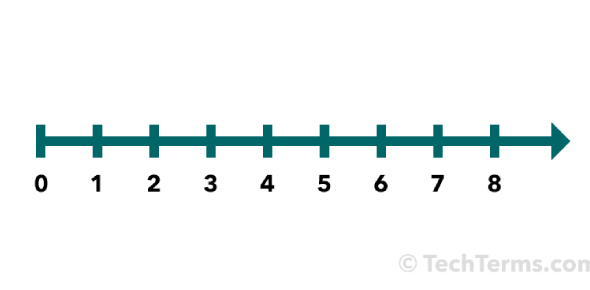# Whole Number Quiz

20 Questions | Total Attempts: 5905SettingsThis is a timed whole number quiz.

• 1.
True/False An even number plus an odd number is always an odd number.
• A.

True

• B.

False

• 2.
True/False An odd number minus an even number is always an even number.
• A.

True

• B.

False

• 3.
2378 + 1321 =
• A.

3679

• B.

3099

• C.

3659

• D.

3699

• E.

None of these

• 4.
1876 + 8 =
• A.

1874

• B.

1884

• C.

1076

• D.

1878

• E.

None of these

• 5.
516 + 29 =
• A.

545

• B.

535

• C.

806

• D.

525

• E.

None of these

• 6.
395 - 142 =
• A.

243

• B.

252

• C.

242

• D.

253

• E.

None of these

• 7.
2342 - 331 =
• A.

2011

• B.

2042

• C.

2111

• D.

2311

• E.

None of these

• 8.
507 - 63 =
• A.

444

• B.

544

• C.

443

• D.

545

• E.

None of these

• 9.
5419 - 170 =
• A.

5369

• B.

5349

• C.

5249

• D.

5449

• E.

None of these

• 10.
1090 - 805 =
• A.

290

• B.

210

• C.

295

• D.

285

• E.

None of these

• 11.
521 x 24 =
• A.

13026

• B.

13126

• C.

12504

• D.

12404

• E.

None of these

• 12.
150 x 3 =
• A.

45

• B.

35

• C.

350

• D.

650

• E.

None of these

• 13.
240 x 32 =
• A.

120

• B.

768

• C.

668

• D.

7248

• E.

None of these

• 14.
96 x 57 =
• A.

1152

• B.

5472

• C.

872

• D.

5372

• E.

None of these

• 15.
203 x 4 =
• A.

812

• B.

8012

• C.

612

• D.

807

• E.

None of these

• 16.
294 ÷ 7 =
• A.

40 R 4

• B.

42

• C.

40 R 2

• D.

32

• E.

None of these

• 17.
6000 ÷ 4 =
• A.

150

• B.

1200

• C.

1400

• D.

1500

• E.

None of these

• 18.
9021 ÷ 3 =
• A.

37

• B.

307

• C.

3007

• D.

3070

• E.

None of these

• 19.
184 ÷ 20 =
• A.

94

• B.

90

• C.

9 R 4

• D.

90 R 4

• E.

None of these

• 20.
306 ÷ 30 =
• A.

12

• B.

102

• C.

101

• D.

192

• E.

None of these

Related TopicsBack to top